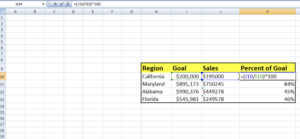# Percent to Goal Calculator

Measuring progress as a percentage of the goal can be a valuable tool for tracking progress in any objective you aim to achieve.

$$\dfrac{progress}{goal} × 100$$

Whether you have a monthly sales target, a specific distance to run each week, or a fundraising goal to meet, assessing your progress as a percentage of the overall goal can provide a valuable perspective on your progress. In this way, the Percent to Goal Calculator can help you stay on track and monitor your progress towards your goals.

## Percent to Goal Formula:

You can use the percent to Goal formula to determine your progress towards a specific target or forecast. This involves dividing your actual outcome by your target or forecast. The result will be a percentage indicating how much progress you have made towards your objective.

To calculate percent to goal, you need to divide the actual amount achieved by the goal amount and then multiply it by 100.

Percentage to $$Goal = \dfrac{Progress}{Goal} × 100$$

## How to Calculate Percent to Goal

To begin tracking your progress towards a goal, first determine the specific objective you want to achieve.

For instance, if you want to raise $2,500, your goal would be to collect that amount in donations. Next, measure your progress toward your goal. For example, if you received a$100 donation from one donor and a $450 donation from another, you would have$550 raised towards your $2,500 goal. To determine the percentage of progress you have made towards your goal, divide your progress by your target. For this example, divide$550 by \$2,500 to get 0.22.

Finally, multiply the result by 100 to convert it to a percentage. Here we will multiply 0.22 by 100 to get 22 percent.

## Calculating Percent of Goal in Excel

When asked to calculate a percentage of a goal, it means comparing actual performance to a predetermined target. The calculation involved in this process is straightforward: divide the actual outcome by the goal. The result will be a percentage that indicates how much of the goal has been accomplished.

For example, if your goal is to sell 50 movie tickets, and you manage to sell 10, your percentage of the goal would be 20 percent $$\frac{10}{50}$$.

In the table, you can see a list of America States with columns for both goals and sales. Note that the formula in cell K10 divides the value in the Sales column by the value in the Goal column and multiplies the result by 100, resulting in a percentage of the goal in column K.## FAQs

Calculate the percent to goal if the progress value is 8 and the goal is 12?

To calculate this, divide 8 by 12 and multiply the result by 100. The percentage to goal would be about 66.67%.

What is the percent to goal if the progress value is 50 and the goal is 1000?

This means you divide 50 by 1000 and multiply the result by 100 to get the percentage. In that case, the percentage to goal would be 5%.

What is the percent to goal if the progress value is 75 and the goal is 200?

To calculate this, divide 75 by 200 and multiply by 100. then the percent to goal would be 37.5%.